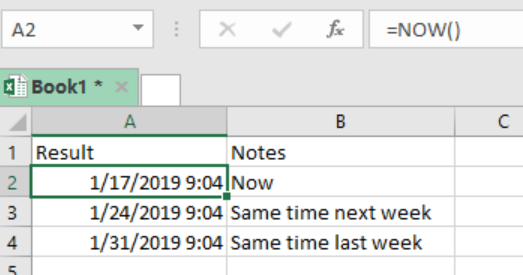Get instant live expert help with Excel or Google Sheets“My Excelchat expert helped me in less than 20 minutes, saving me what would have been 5 hours of work!”

#### Post your problem and you’ll get Expert help in seconds.

Your message must be at least 40 characters
Our professional Expert are available now. Your privacy is guaranteed.

# Excel NOW Function

We can use the Excel NOW function to get the current date and time in a given cell. This time and date will be automatically updated each time the worksheet is either changed or opened. To learn how to use the Excel NOW function, read this post to the end.

##Figure 1. Final result

## General syntax of the formula

`=NOW ()`

## Understanding the Excel NOW function

• This function will return the current date and time, which updates itself automatically every time the sheet is changed or opened.
• The function does not need any arguments. All you need is to use empty parentheses.
• To format the value returned by the NOW function either as a date or a date with time, you need to apply number format.
• If you want to recalculate and update the time and date value, you can use the F9 key to force it.

## Note

If you want to get a time and date that won’t change every time the sheet is opened or updated, enter the current time using the keyboard shortcut, Ctrl + Shift + :

Most of the time, the problem you will need to solve will be more complex than a simple application of a formula or function. If you want to save hours of research and frustration, try our live Excelchat service! Our Excel Experts are available 24/7 to answer any Excel question you may have. We guarantee a connection within 30 seconds and a customized solution within 20 minutes.

### Did this post not answer your question? Get a solution from connecting with the expert.Another blog reader asked this question today on Excelchat:
Solution examplesI would like my result cell to display 1 of 5 values: If my referenced cell value is less than 2 then the result cell should display 2. (values 0 thru 1.99 = 2) but if my referenced cell value is greater than 2 but less than 2.99 then the new cell should display 3 (values 2 thru 2.99 = 3) but if my referenced cell value is greater than 3 but less than 4.99 then the new cell should display 5 (values 3 thru 4.99 = 5) but if my referenced cell value is greater than 5 but less than 7.5 then the new cell should display 7.5 (values 5 thru 7.5 = 7.5)
Solved by S. Q. in 15 minsi want to insert a multiplication formula in a cell if some percentage value is entered in another cell of same row. for eg. if i put a formula in G7 cell (=G2*e7) but it should be enabled or activated if i put some data entry in f7 cell Excel
Solved by Z. D. in 20 minsDo I have to pay for a solution ? I just wasted time with another help desk that asked for \$46 for an answer. If that is the case, thanks anyway.
Solved by X. U. in 18 minsi would like the cell to become red if the date in the cell exceeds "NOW"
Solved by K. F. in 20 minsI have =NOW() in A2, and deadline dates in Column K What I am looking for is the correct conditional formatting, to give me a 1. yellow highlight when within 7 days 2. red when the deadline has passed
Solved by D. D. in 23 mins## Subscribe to Excelchat.coAnother blog reader asked this question today on Excelchat: